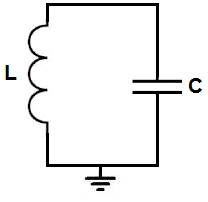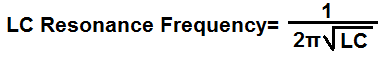﻿ LC Resonance Calculator ﻿# LC Resonance CalculatorEnter the Frequency Desired Hz (hertz) KHz (kilohertz) MHz (megahertz)

C:
L:

﻿

The LC resonance frequency calculator is a calculator that computes the resonant frequency that is created from a single inductor and a single capacitor combined together.

The LC resonant circuit is composed of 1 inductor and 1 capacitor.

Just like an RC circuit, oscillations are produced. RC circuits have a frequency according to the formula, frequency= 1/RC.

LC circuits have a frequency according to the formula, frequency= 1/2πLC.

LC circuits produce oscillations through the continual discharging and recharging of a capacitor.

LC circuits can be incorporated into many different electronic components such as op amps, transistors, logic chips. Regardless of which component it is used in, it serves the same function and its composition of one inductor and one capacitor remains unchanged. Therefore, this calculator can be used for any LC circuit. The formula for calculating the frequency of the LC circuit will remain unchanged.

This calculator uses a variety of inductor and capacitor values. This calculator tries to take into account that for breadboarding purposes, large capacitor values are usually very hard to find. Components easily obtained for capacitors are values from 1pF to 4700μF. Therefore, this calculator only uses large capacitor values (up to 1 farad) for very small frequencies such as from 1Hz to 100Hz. Other than this, large capacitor values are avoided due to the fact of not easy accessibility.

Inductor values are most commonly used in the nanohenries to microhenries range. Just like with capacitors, large values for inductors such as in the millihenry range are generally avoided due to the fact that capacitors in the millifarads range are hard to obtain and not easily accessible. In fact, in this calculator, frequency values only from 1-2Hz utilize inductors in the millihenry range. The rest of inductors are smaller, which is much more easily accessible.

In general, low frequencies require very large capacitor and inductor values. For very small frequency signals, such as a few hertz in frequency, very large values are needed for the inductor and capacitor. As the value of the frequency increases, the values of the capacitors and inductor get much smaller. At very high frequencies, such as in the megahertz range, very small inductor and capacitors values are used. For frequencies 1MHz to 99.99MHz, capacitors in the picofarads range and an inductor in the microhenry range are used. For frequencies 100MHz or greater, capacitors in the picofarads and an inductor in the nanohenries is used.

This calculator can handle frequencies up to a few gigahertz. After this frequency, the inductors values fall too low and inductors so small in value are hard to find, thus making the circuit uneasily accessible to build. But normally circuits don't deal with frequencies nearly so high, so this calculator can give very practical components that are easy to find.

To use this calculator, a user simply has to enter the frequency desired. The frequency can be entered in hertz (Hz), kilohertz (KHz), and megahertz (MHz). The calculator will then compute the value of the inductor and capacitor.

As stated, LC circuits may be built with an op amp, a transistor, or inverter logic chip, but its composition of an inductor and capacitor is the same for each case. Therefore, this formula and calculator will work for any arrangement.

Related Resources

﻿